# Summary

Combinatorial therapies is one of the major strategies for improving treatment efficacy in treating cancer. High-throughput drug combination screening has the advantage of assaying a large collection of chemical compounds in search for promising drug pairs. Studies that utilizes drug combination screening have generated dynamic dose-response profiles that allow researchers to quantify the degree of drug-drug interactions at an unprecedented level. SynergyFinder R package is a software tool to analyze such pre-clinical drug combination data sets. It provides efficient implementations for

1. all the popular synergy scoring models, including HSA, Loewe, Bliss, and ZIP to quantify the degree of drug synergy;
2. higher order drug combination data analysis and synergy landscape visualization for unlimited number of drugs in a combination;
3. statistical analysis of drug combination synergy and sensitivity with confidence intervals and p-values;
4. synergy barometer for harmonizing multiple synergy scoring methods to provide a consensus metric of synergy;
5. evaluation of synergy and sensitivity simultaneously to provide an unbiased interpretation of the clinical potential of the drug combinations.

To facilitate the use of the R package for the drug discovery community, we also provide a web server at https://synergyfinderplus.org/ as a user-friendly interface to enable a more flexible and versatile analysis of drug combination data. In the web application, in addition to the functions in R package, we provide the annotation of drugs and cell lines that are tested in an experiment, including their chemical information, targets and signaling network information.

# 1 Installation

Note: We recommend to install Bioconductor >= 3.13 and SynergyFinder >= 3.0.1 to access the new features described in this vignette.

To install the SynergyFinder package from Bioconductor, start R (>= 4.0) and then enter:

if (!requireNamespace("BiocManager", quietly = TRUE))
install.packages("BiocManager")

BiocManager::install("synergyfinder")

library(synergyfinder)

# 2 Input data

synergyfinder requires a data frame that describes the drug combination data set. The dose-response data is represented as a long table where each row represent one observation in the dose-response matrix.

The input table must contain the following columns (The column naming styles used by the old versions of synergyfinder or DrugComb, i.e. ‘Alternative Column names’ are accepted):

Required Columns Alternative Column names Description
block_id PairIndex, BlockId Identifier for the drug combination blocks.
drug1 Drug1, drug_row, DrugRow Name of the first tested drug.
drug2 Drug2, drug_col, DrugCol Name of the second tested drug.
conc1 Conc1, conc_row, ConcRow Concentration of first tested drug.
conc2 Conc2, conc_col, ConcCol Concentration of second tested drug.
response Response, inhibition, Inhibition Cell response to the drug treatment (%inhibition or %viability).
conc_unit ConcUnit Unit of concentration for drugs. This column could be replaced by multiple separated columns for each tested drugs (see table below), while different unit was used for measuring the concentrations.

There are several optional columns available for the higher-order drug combination data sets or the data sets using different concentration units for drugs.

Optional Columns Alternative Column names Description
conc_unit1 conc_r_unit Unit of concentration for the first drug. Used if the concentration units are not identical across the drugs tested in one block.
conc_unit2 conc_c_unit Unit of concentration for the second drug. Used if the concentration units are not identical across the drugs in test one block.
drug[n] Name of the n_th_ tested drug. For example, “drug3” for the third tested drug. Used for higher-order drug combination data point.
conc[n] Concentration of n_th_ tested drug. Used for higher-order drug combination data point.
conc_unit[n] Unit of concentration for n_th drug. Used if the concentration units are not identical across the drugs in test one block.

Note:

1. The duplicated concentration combinations in one block (with the same “block_id”) will be treated as replicates.
2. There is no restriction on the number of drug combinations (blocks) for the input file. The data should however, contain at least three concentrations for each drug in each block, so that sensible synergy scores can be calculated.
3. synergyfinder allows for missing values in the dose-response matrix. The missing value will be automatically imputed by mice R package.

SynergyFinder provides an example input data (mathews_screening_data) in the package, which is extracted from a published drug combination screening study for the treatment of diffuse large B-cell lymphoma (DLBCL) . The example input data contains two representative drug combinations (ibrutinib & ispinesib and ibrutinib & canertinib) for which the %viability of a cell line TMD8 was assayed using a 6 by 6 dose matrix design. To load the example data, please run:

data("mathews_screening_data")
#>   block_id  drug_row  drug_col conc_r  conc_c  response conc_r_unit conc_c_unit
#> 1        1 ispinesib ibrutinib   2500 50.0000  7.802637          nM          nM
#> 2        1 ispinesib ibrutinib   2500 12.5000  6.831317          nM          nM
#> 3        1 ispinesib ibrutinib   2500  3.1250 15.089589          nM          nM
#> 4        1 ispinesib ibrutinib   2500  0.7812 24.503885          nM          nM
#> 5        1 ispinesib ibrutinib   2500  0.1954 38.043076          nM          nM
#> 6        1 ispinesib ibrutinib   2500  0.0000 45.790634          nM          nM

# 3 Reshaping and pre-processing

We provide a function ReshapeData to reshape and pre-process the input data for further analysis:

Important parameters:

• data_type: User must specify the type for the observations in column “response” in the input data. Its value must be either “viability” meaning the %viability of cell or “inhibition” indicating %inhibition of the cell growth;
• impute: Impute missing values in response data by calling function mice::mice. If input matrix contains missing values, the function will automatically perform the imputation with the selected imputation method. The parameter impute_method is used to define the method in imputation. The default method (when impute_method = NULL) is predictive mean matching (pmm). Please check the doc for mice function in mice R package for more available methods;
• noise: Add small random number to response data to avoid the error caused by exact same response values. We recommend to set it as TRUE. The parameter seed is used to set the random seed to make the result reproducible.
res <- ReshapeData(
data = mathews_screening_data,
data_type = "viability",
impute = TRUE,
impute_method = NULL,
noise = TRUE,
seed = 1)

The output data is a list containing following components:

• drug_pairs: A data frame contains the name of all the tested drugs, concentration unit, block IDs and a logical column “replicate” to indicate whether there are replicates in the corresponding block.
• response A data frame contains the columns:
• “block_id” - the identifier for blocks;
• “concX” - concentrations for drugs from input data;
• “response_origin” - response values from input data;
• “response” - %inhibition value for downstream analysis. It has been added the random noise and imputed missing values.
str(res)
#> List of 2
#>  $drug_pairs: tibble [2 × 7] (S3: tbl_df/tbl/data.frame) #> ..$ block_id  : int [1:2] 1 2
#>   ..$drug1 : chr [1:2] "ispinesib" "canertinib" #> ..$ drug2     : chr [1:2] "ibrutinib" "ibrutinib"
#>   ..$conc_unit1: chr [1:2] "nM" "nM" #> ..$ conc_unit2: chr [1:2] "nM" "nM"
#>   ..$input_type: chr [1:2] "viability" "viability" #> ..$ replicate : logi [1:2] FALSE FALSE
#>  $response : tibble [72 × 5] (S3: tbl_df/tbl/data.frame) #> ..$ block_id       : int [1:72] 1 1 1 1 1 1 1 1 1 1 ...
#>   ..$conc1 : num [1:72] 2500 2500 2500 2500 2500 2500 625 625 625 625 ... #> ..$ conc2          : num [1:72] 50 12.5 3.125 0.781 0.195 ...
#>   ..$response : num [1:72] 92.2 93.2 84.9 75.5 62 ... #> ..$ response_origin: num [1:72] 7.8 6.83 15.09 24.5 38.04 ...

# 4 Synergy and sensitivity analysis

## 4.0 Baseline Correction

Typically, the models for estimating synergy or sensitivity of drug combinations expect that the drug’s effect to be expressed as continuous values ranged between 0 and 1 (or between 0% and 100%). However, in practice the readouts from the drug combination screening could be negative. In this cases, the technical error might be introduced in the dose-response data. The base line correction function is designed to adjust the “baseline” of the dose-response data closer to 0. Following is the main steps for the process of “baseline correction”:

1. Extract all the single drug treatment (monotherapy) dose-response data from the combination.
2. Fit the four-parameter log logistic model for each monotherapy with the data table from step 1.
3. Pick the minimum fitted response value from all the models fitted in step 2 as “baseline”.
4. Adjusted response value = observed response value - ((100 – observed response value) / 100 * baseline).

By setting the value of the parameter correct_baseline while calling function CalculateSynergy or CalculateSensitivity, user could chose:

• “non” - do not correct base line;
• “part” - correct base line but only adjust negative response values in matrix;
• “all” - correct base line with adjusting all values in matrix.

## 4.1 Drug synergy scoring

The synergistic effect can be determined as the excess of observed effect over expected effect calculated by a reference models (synergy scoring models). All of the models make different assumptions regarding the expected effect. Currently, 4 reference models are available in SynergyFinder.

• Highest Single Agent (HSA)  states that the expected combination effect equals to the higher effect of individual drugs:

$y_{HSA} = max(y_1, y_2)$ where $$y_1$$ and $$y_2$$ are the monotherapy effect of combined drug 1 and drug 2

• Bliss model  assumes a stochastic process in which two drugs exert their effects independently, and the expected combination effect can be calculated based on the probability of independent events as:

$y_{Bliss} = y_1 + y_2 - y_1 \cdot y_2$ where $$y_1$$ and $$y_2$$ are the monotherapy effect of combined drug 1 and drug 2

• Loewe additivity model  defines the expected effect $$y_{Loewe}$$ as if a drug was combined with itself, i.e. $$y_{Loewe} = y_1(x_1 + x_2) = y_2(x_1 + x_2)$$. Unlike the HSA and the Bliss independence models, which give a point estimate using different assumptions, the Loewe additivity model considers the dose-response curves of individual drugs. The expected effect $$y_{Loewe}$$ must satisfy:

$\frac {x_1}{\chi_{Loewe}^1} + \frac{x_2}{\chi_{Loewe}^2} = 1$

where $$x_{1,2}$$ are drug doses and $$\chi_{Loewe}^1,\ \chi_{Loewe}^2$$ are the doses of drug 1 and 2 alone that produce $$y_{Loewe}$$. Using 4-parameter log-logistic (4PL) curves to describe dose-response curves the following parametric form of previous equation is derived:

$\frac {x_1}{m_1(\frac{y_{Loewe}-E_{min}^1}{E_{max}^1 - y_{Loewe}})^{\frac{1}{\lambda_1}}} + \frac{x_2}{m_2(\frac{y_{Loewe}-E_{min}^2}{E_{max}^2 - y_{Loewe}})^{\frac{1}{\lambda_2}}} = 1$ where $$E_{min}, E_{max}\in[0,1]$$ are minimal and maximal effects of the drug, $$m_{1,2}$$ are the doses of drugs that produce the midpoint effect of $$E_{min} + E_{max}$$, also known as relative $$EC_{50}$$ or $$IC_{50}$$, and $$\lambda_{1,2}(\lambda>0)$$ are the shape parameters indicating the sigmoidicity or slope of dose-response curves. A numerical nonlinear solver can be then used to determine $$y_{Loewe}$$ for ($$x_1$$, $$x_2$$).

• Zero Interaction Potency (ZIP)  calculates the expected effect of two drugs under the assumption that they do not potentiate each other, i.e. both the assumptions of the Loewe model and the Bliss model are met:

$y_{ZIP} = \frac{(\frac{x_1}{m_1}) ^ {\lambda_1}}{(1 + \frac{x_1}{m_1})^{\lambda_1}}+\frac{(\frac{x_2}{m_2})^{\lambda_2}}{(1 + \frac{x_2}{m_2})^{\lambda_2}} - \frac{(\frac{x_1}{m_1})^{\lambda_1}}{(1 + \frac{x_1}{m_1})^{\lambda_1}} \cdot \frac{(\frac{x_2}{m_2})^{\lambda_2}}{(1 + \frac{x_2}{m_2})^{\lambda_2}}$ where $$x_{1, 2}, m_{1, 2}, and\ \lambda_{1, 2}$$ are defined in the Loewe model.

For the input data without replicates, the statistical analysis for synergy scores of the whole dose-response matrix is estimated under the null hypothesis of non-interaction (zero synergy score). Suppose that $$S_1, S_2, ...S_n$$ are the synergy scores for all the concentration combinations (excluding monotherapies) for the dose-response matrix with mean of $$\bar s$$. The p-value is:

$p = exp(-0.717z - 0.416z^2),\ where\ z = abs(\bar s')/\sqrt{\frac{1}{n - 1}\sum_{i = 1}^n(s_i' - \bar s')^2}$

The function CalculateSynergy is designed to calculate the synergy scores. Important parameters are:

• data: The R object generated by function ReshapeData;
• method: The reference model for synergy score calculation. Parameter Emin and Emax are used to set the corresponding parameters in the 4 parameter log-logistic model which will be fitted to calculate “ZIP” and “Loewe” synergy scores;
• correct_baseline: Whether to adjust base line of response matrix.
res <- CalculateSynergy(
data = res,
method = c("ZIP", "HSA", "Bliss", "Loewe"),
Emin = NA,
Emax = NA,
correct_baseline = "non")

The output adds one data frame “synergy_scores” to the input R object. It contains:

• “block_id” - The identifier for drug combination blocks;
• “concX” - The concentration for combined drugs;
• “ZIP_ref”, “HSA_ref”, “Bliss_ref”, and “Loewe_ref” - The reference additive effects calculated from corresponding models;
• “ZIP_synergy”, “HSA_synergy”, “Bliss_synergy” and "Loewe_synergy - The synergy scores calculated from corresponding models;
• “ZIP_fit” - The fitted %inhibition for combinations which is used to calculate the ZIP synergy score.

The mean of synergy scores for the combination matrix (excluding the monotherapy observations) and the p-values for them are added to the “drug_pairs” table in the input R object.

res$drug_pairs #> # A tibble: 2 × 15 #> block_id drug1 drug2 conc_unit1 conc_unit2 input_type replicate #> <int> <chr> <chr> <chr> <chr> <chr> <lgl> #> 1 1 ispinesib ibrutinib nM nM viability FALSE #> 2 2 canertinib ibrutinib nM nM viability FALSE #> # … with 8 more variables: ZIP_synergy_p_value <chr>, #> # HSA_synergy_p_value <chr>, Bliss_synergy_p_value <chr>, #> # Loewe_synergy_p_value <chr>, ZIP_synergy <dbl>, HSA_synergy <dbl>, #> # Bliss_synergy <dbl>, Loewe_synergy <dbl> str(res$synergy_scores)
#> tibble [72 × 12] (S3: tbl_df/tbl/data.frame)
#>  $block_id : int [1:72] 1 1 1 1 1 1 1 1 1 1 ... #>$ conc1        : num [1:72] 2500 2500 2500 2500 2500 2500 625 625 625 625 ...
#>  $conc2 : num [1:72] 50 12.5 3.125 0.781 0.195 ... #>$ ZIP_fit      : num [1:72] 93.3 92.3 82.7 75.2 57.3 ...
#>  $ZIP_ref : num [1:72] 79.4 79.4 67.9 37.2 37.2 ... #>$ ZIP_synergy  : num [1:72] 13.9 12.9 14.8 38.1 20.1 ...
#>  $HSA_ref : num [1:72] 71.3 54.2 54.2 54.2 54.2 ... #>$ HSA_synergy  : num [1:72] 20.89 38.96 30.7 21.29 7.75 ...
#>  $Bliss_ref : num [1:72] 86.9 78.6 73.1 45.9 52.5 ... #>$ Bliss_synergy: num [1:72] 5.34 14.54 11.85 29.6 9.47 ...
#>  $Loewe_ref : num [1:72] 62.3 62.3 62.3 62.3 -14.9 ... #>$ Loewe_synergy: num [1:72] 29.9 30.8 22.6 13.2 76.9 ...

## 4.2 Sensitivity scoring

SynergyFinder mainly calculates 3 sensitive scores: relative IC50 and relative inhibition (RI) for single drug treatment, and combination sensitivity score (CSS) for drug combinations.

• Relative IC50: SynergyFinder extracts the single drug treatment response data from the combination matrix to fit the 4-parameter log-logistic curve:

$y = y_{min} + \frac{y_{max} - y_{min}}{1 + 10^{\lambda(log_{10}IC_{50} - x')}}$ where $$y_{min}, y_{max}$$ are minimal and maximal inhibition and $$x' = log_{10}x$$

• RI: RI is the abbreviation of “relative inhibition”. It is the proportion of the area under the dose-response curve (fitted by four parameter log logistic model), to the maximal area that one drug can achieve at the same dose range (Figure 1). For example, RI of 40 suggests that the drug is able to achieve 40% of the maximal of inhibition (e.g. a positive control where the cell is 100% inhibited in each dose that in the range). RI is comparable between different scenarios, even for the same drug-cell pair but tested in different concentration ranges.
knitr::include_graphics("./ri.png")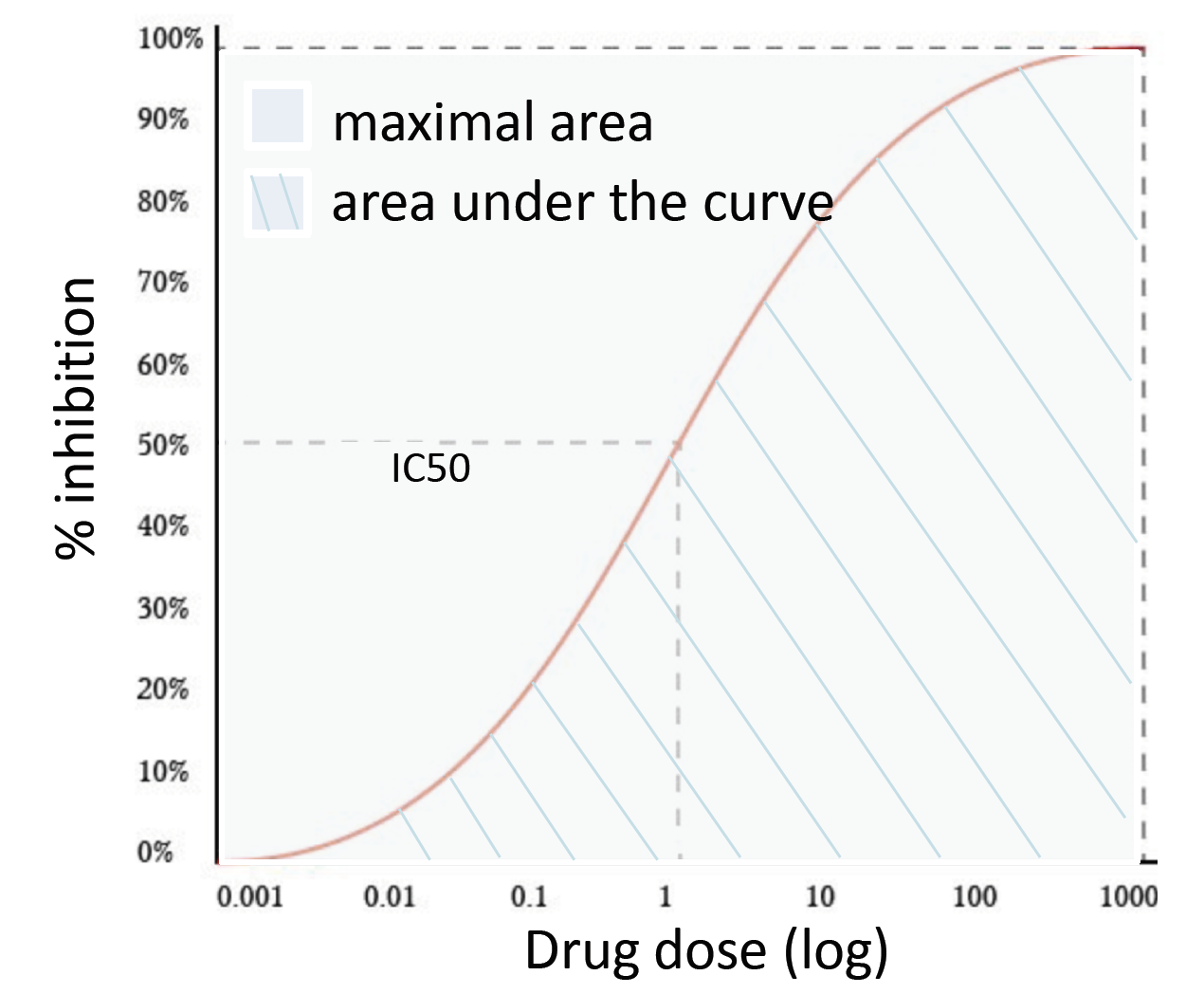Figure 1. Concept of RI

• CSS: CSS is the abbreviation of “Combination Sensitivity Score”. It is derived using relative IC50 values of compounds and the area under their dose-response curves. CSS of a drug combination is calculated such that each of the compounds (background drug) is used at a fixed concentration (its relative IC50) and another is at varying concentrations (foreground drug) resulting in two CSS values, which are then averaged. Each drug’s dose-response is modeled using 4-parameter log-logistic curve. The area under the log-scaled dose-response curve (AUC) is then determined according to:

$AUC = \int_{c_1}^{c_2}y_{min} + \frac{y_{max}-y_{min}}{1 + 10^{\lambda(log_{10}IC_{50}-x')}}dx'$ where $$[c_1, c_2]$$ is the concentration range of the foreground drug tested.

The function CalculateSensitivity is designed to calculate the synergy scores. Important parameters are:

• data: The R object generated by function ReshapeData;
• correct_baseline: Whether to adjust base line of response matrix
res <- CalculateSensitivity(
data = res,
correct_baseline = "non"
)

Following sensitivity scores are added to the “drug_pairs” table in the input R object:

• “ic50_x”: The relative IC50 for drug x;
• “ri_x”: The RI for drug x;
• “cssx_ic50y”: The css for drug x while drug y is at its relative IC50;
• “css”: The CSS for the combination.
sensitive_columns <- c(
"block_id", "drug1", "drug2",
"ic50_1", "ic50_2",
"ri_1", "ri_2",
"css1_ic502", "css2_ic501", "css")
res\$drug_pairs[, sensitive_columns]
#> # A tibble: 2 × 10
#>   block_id drug1    drug2  ic50_1 ic50_2  ri_1  ri_2 css1_ic502 css2_ic501   css
#>      <int> <chr>    <chr>   <dbl>  <dbl> <dbl> <dbl>      <dbl>      <dbl> <dbl>
#> 1        1 ispines… ibrut…  2500    2.74  60.0  27.0       85.9      82.8   84.3
#> 2        2 canerti… ibrut…   973.   1.44 -48.0  45.2      -35.0      -6.99 -21.0

# 5 Visualization

## 5.1 Dose-response curve

The PlotDoseResponseCurve function will plot the dose-response curve fitted by 4-parameter log logistic function for selected drug. Important parameters are:

• data: The R object generated by function ReshapeData;
• plot_block: Select the block from which to extract the monotherapy dose-response data;
• drug_indes: Select the drug from the combination to draw the dose-response curve. Available values are 1, 2, 3, … to indicates the drug 1, drug 2, drug 3, … in the combination.

This function will return an object of class recordedplot. User could use replayPlot to plot it. User could modify the parameter plot_setting to control the themes of some items in the plot.

for (i in c(1, 2)){
PlotDoseResponseCurve(
data = res,
plot_block = 1,
drug_index = i,
plot_new = FALSE,
record_plot = FALSE
)
}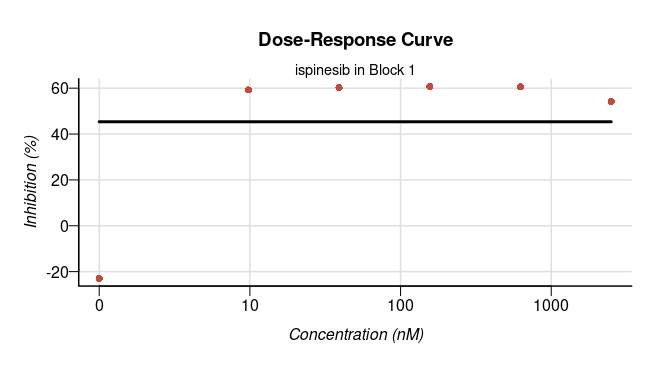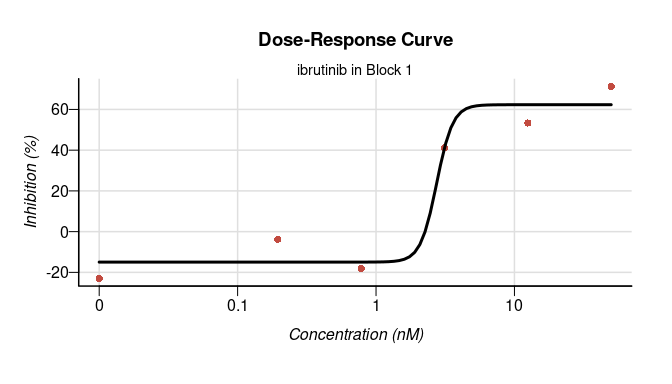If parameter record_plot is set as TRUE, the function will return a plot object recorded by recordPlot.

## 5.2 Two-drug combination visualization

SynergyFinder provides 3 types (Heatmap, 2D contour plot, or 3D surface) of plots to visualize dose-response map or synergy map for two-drug combinations.

From the plots for dose-response landscape, user can assess the therapeutic significance of the combination, e.g. by identifying the concentrations at which the drug combination can lead to a maximal effect on cancer inhibition.

The landscape of drug interaction scoring is very informative when identifying the specific dose regions where a synergistic or antagonistic drug interaction occurs.

Important parameters shared by all the three functions are:

• data: The R object generated by function ReshapeData or CalculateSynergy;
• plot_block: Select the block from which to extract the two-drug combination matrix;
• drugs: Select two drugs for plot from the combination. Available values are 1, 2, 3, … to indicates the drug 1, drug 2, drug 3, … in the combination;
• plot_value: Select the value for plots. The available values are:
• “response_origin” The original response value in input data. It might be % inhibition or %viability;
• “response” The % inhibition after pre-process by function ReshapeData;

If the data is the output from CalculateSynergy, following values are also available:

• “ZIP_ref”, “Bliss_ref”, “HSA_ref”, or “Loewe_ref” The reference additive effects calculated by ZIP, Bliss, HSA or Loewe model, respectively;
• “ZIP_synergy”, “Bliss_synergy”, “HSA_synergy”, “Loewe_synergy” The synergy score calculated by ZIP, Bliss, HSA or Loewe model, respectively;
• “ZIP_fit” The response fitted by ZIP model.
• dynamic: Whether to plot a dynamic plot or static plot. Dynamic plots are generated with plotyly. It could be used on interactive platforms, such as R Shiny or Rmarkdown.
• summary_statistic: Select the summary statistics for all the plot_value in whole combination matrix. It will be shown as the subtitle in the plots. If it is NULL, no statistics will be printed. User can specify one or more values listed below:
• “mean” Mean value for all the responses or synergy scores in the matrix and the p-value if it is valid;
• “median” Median value for all the responses or synergy scores in the matrix;
• “quantile_90” 90% quantile. User could change the number to print different sample quantile. For example “quantile_50” equal to median.

Following shows the examples for dose-response and ZIP synergy landscape.

### 5.2.1 Heatmap

If dynamic is set as FALSE, this function will return a ggplot object. User could use + theme() to adjust the plot theme.

Plot2DrugHeatmap(
data = res,
plot_block = 1,
drugs = c(1, 2),
plot_value = "response",
dynamic = FALSE,
summary_statistic = c("mean",  "median")
)

Plot2DrugHeatmap(
data = res,
plot_block = 1,
drugs = c(1, 2),
plot_value = "ZIP_synergy",
dynamic = FALSE,
summary_statistic = c( "quantile_25", "quantile_75")
)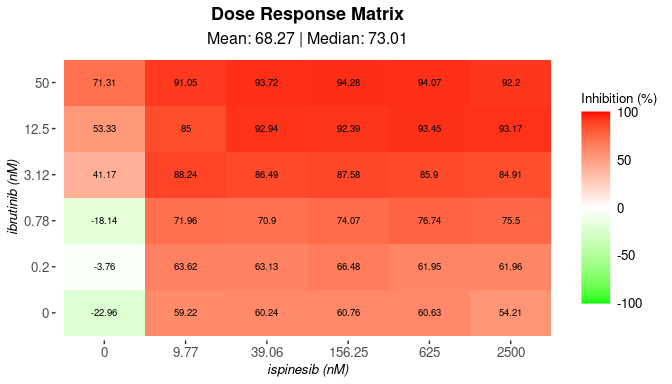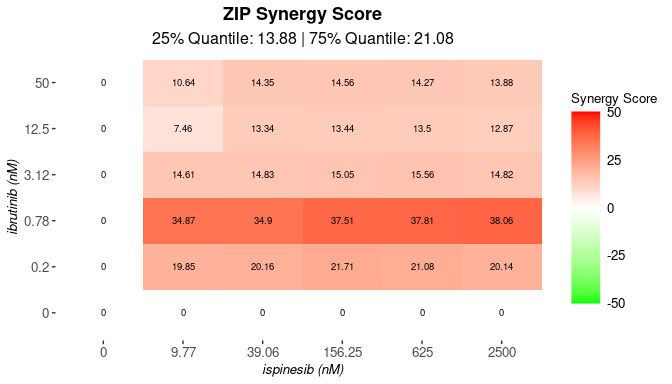### 5.2.2 2D contour plot

If dynamic is set as FALSE, this function will return a ggplot object. User could use + theme() to adjust the plot theme.

Plot2DrugContour(
data = res,
plot_block = 1,
drugs = c(1, 2),
plot_value = "response",
dynamic = FALSE,
summary_statistic = c("mean", "median")
)
Plot2DrugContour(
data = res,
plot_block = 1,
drugs = c(1, 2),
plot_value = "ZIP_synergy",
dynamic = FALSE,
summary_statistic = c("quantile_25", "quantile_75")
)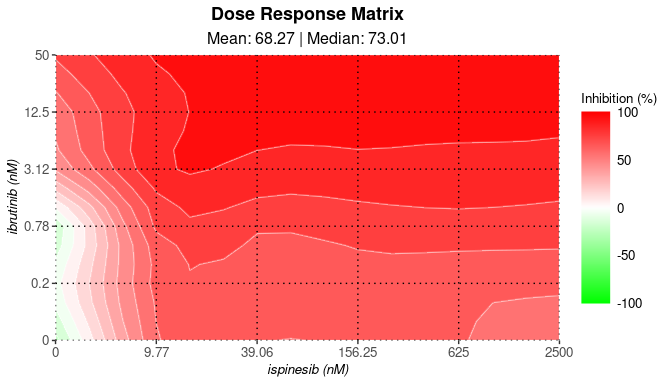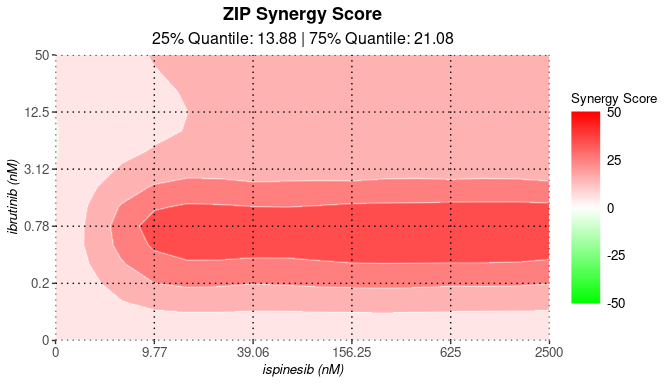### 5.2.3 3D surface plot

If parameter dynamic is set as FALSE, this function will return an object of class recordedplot. User could use replayPlot to plot it.

Plot2DrugSurface(
data = res,
plot_block = 1,
drugs = c(1, 2),
plot_value = "response",
dynamic = FALSE,
summary_statistic = c("mean", "quantile_25", "median", "quantile_75")
)
Plot2DrugSurface(
data = res,
plot_block = 1,
drugs = c(1, 2),
plot_value = "ZIP_synergy",
dynamic = FALSE,
summary_statistic = c("mean", "quantile_25", "median", "quantile_75")
)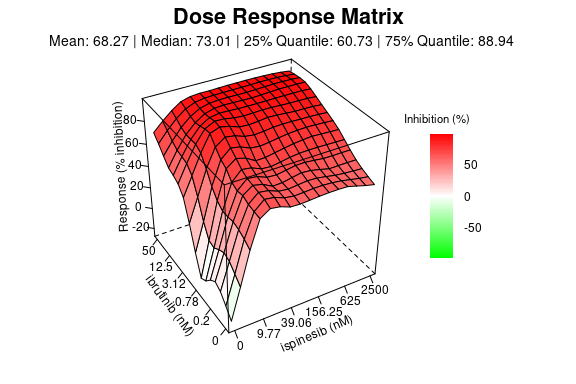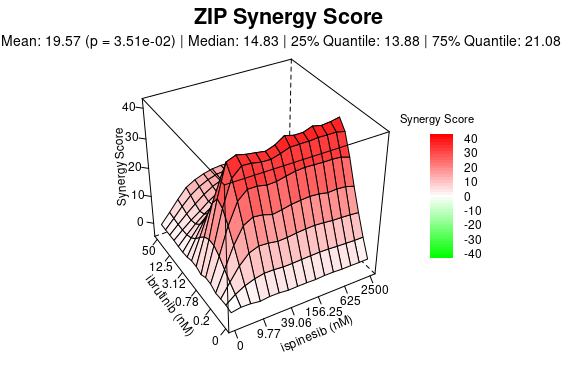It is recommend to plot the 3D surface in dynamic (dynamic=TRUE) way, with which user could adjust the view point in an interactive way to show the surface at the best angle.

## 5.3 Plotting wrapper for two-drug combination

SynergyFinder provides two plotting wrapper for two-drug combination dose-response visualization (PlotDoseResponse) and synergy score visualization (PlotSynergy).

The function PlotDoseResponse combined the dose-response curve and dose-response heatmap in one figure. In addition to the parameters inherited from PlotDoseResponseCurve and Plot2DrugHeatmap, this function has the parameters for saving the plot as a file, including:

• save_file Whether to save the plot into a file;
• file_type The file format. Default is “pdf”. Available values are “jpeg”, “bmp”, “png”, “tiff”, “pdf”, “svg”;
• file_name The name of the file. If it is NULL the default file names containing drug names and block ID are used.
PlotDoseResponse(
data = res,
block_ids = c(1, 2),
drugs = c(1,2),
save_file = TRUE,
file_type = "png"
)